# NEET Physics Gravitation Questions Solved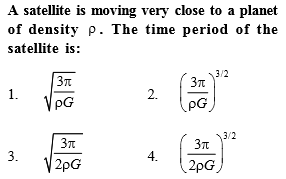$T=\frac{2\mathrm{\pi R}}{v}=\frac{2\mathrm{\pi R}}{\sqrt{\frac{GM}{R}}}=\frac{2{\mathrm{\pi R}}^{3}{2}}}{\sqrt{GM}}\phantom{\rule{0ex}{0ex}}T=\frac{2\pi {R}^{3}{2}}}{\sqrt{G\frac{4}{3}{\mathrm{\pi R}}^{3}\mathrm{\rho }}}=\frac{2{\mathrm{\pi R}}^{3}{2}}}{2\sqrt{\frac{\mathrm{\pi }}{3}\rho G}{R}^{3}{2}}}=\sqrt{\frac{3\mathrm{\pi }}{\rho G}}$

Difficulty Level:

• 46%
• 26%
• 20%
• 10%
Crack NEET with Online Course - Free Trial (Offer Valid Till August 24, 2019)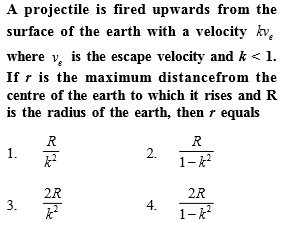$\left(2\right)\phantom{\rule{0ex}{0ex}}{K}_{i}+{U}_{i}={K}_{f}+{U}_{f}\phantom{\rule{0ex}{0ex}}\frac{1}{2}m{\left(k{v}_{e}\right)}^{2}-\frac{GMm}{R}=0-\frac{GMm}{r}\phantom{\rule{0ex}{0ex}}\frac{1}{2}m{\left(k\sqrt{\frac{2GM}{R}}\right)}^{2}-\frac{GMm}{R}=0-\frac{GMm}{r}\phantom{\rule{0ex}{0ex}}\frac{{k}^{2}}{R}-\frac{1}{R}=-\frac{1}{r}\phantom{\rule{0ex}{0ex}}r=\frac{R}{1-{k}^{2}}$

Difficulty Level:

• 15%
• 57%
• 18%
• 12%
Crack NEET with Online Course - Free Trial (Offer Valid Till August 24, 2019)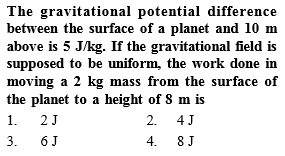Difficulty Level:

• 13%
• 33%
• 23%
• 33%
Crack NEET with Online Course - Free Trial (Offer Valid Till August 24, 2019)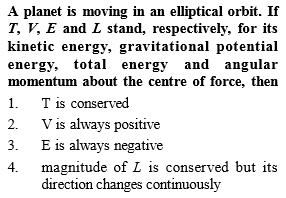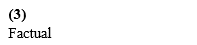Difficulty Level:

• 10%
• 11%
• 34%
• 47%
Crack NEET with Online Course - Free Trial (Offer Valid Till August 24, 2019)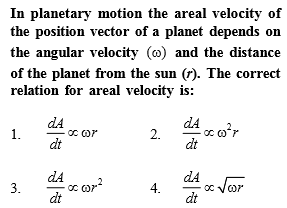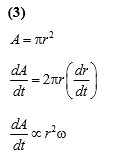Difficulty Level:

• 21%
• 33%
• 42%
• 6%
Crack NEET with Online Course - Free Trial (Offer Valid Till August 24, 2019)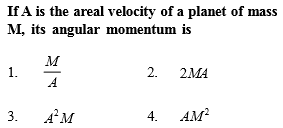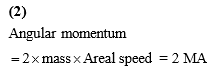Difficulty Level:

• 13%
• 64%
• 19%
• 6%
Crack NEET with Online Course - Free Trial (Offer Valid Till August 24, 2019)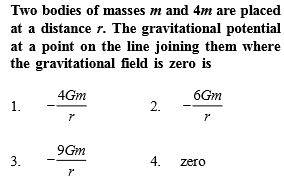Difficulty Level:

• 23%
• 16%
• 38%
• 26%
Crack NEET with Online Course - Free Trial (Offer Valid Till August 24, 2019)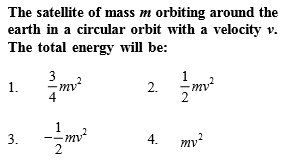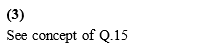Difficulty Level:

• 12%
• 25%
• 57%
• 8%
Crack NEET with Online Course - Free Trial (Offer Valid Till August 24, 2019)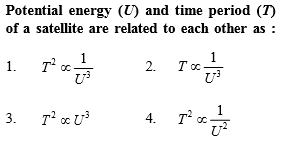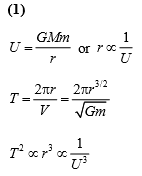Difficulty Level:

• 45%
• 19%
• 27%
• 11%
Crack NEET with Online Course - Free Trial (Offer Valid Till August 24, 2019)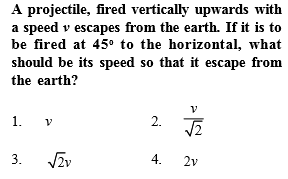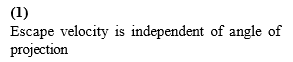Difficulty Level:

• 43%
• 23%
• 31%
• 6%
Crack NEET with Online Course - Free Trial (Offer Valid Till August 24, 2019)